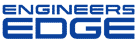# Re: Torque Limits

[ Home ][ Forum Archive ] [ Active Engineering Forum ]

Posted By<" ">B.J. Hughes on May 21, 2002 at 09:21:58:

In Reply to: Torque Limits posted byBrock Presgrove on April 30, 2002 at 09:46:53:

Brock,
This info may be too late, but below is what I use to determine torque for various size screws/bolts...

The formula for torque on a bolt/screw is:
T = K*L*D; where T=tightening torque(lb-in), K=dynamic coefficient of friction (i.e. minimum=.15 (dry-zinc plated)), L=clamp load (lbf) and D=nominal screw diameter (in)

Clamp Load can be found as follows:
Max Clamp Load = (minimum yield strength of material)*(stress area of screw)
Min Clamp Load = (Lmax - (.25*Lmax))

The stress area for a 1"-8 screw is .606 sq-in. The stress area for a 1"-12 screw is .663 sq-in. The yield strength for Grade 8 is 130,000 psi.
Tightening torque range for 1"-8 Grade 8: 738.6-984.8 lb-ft

I hope that this info helps.
Regards,
B.J.

: How do you determine the maximum allowable torque on a bolt? I reviewed the Torque Design page and don't understand how they came up with the values. For a Grade 8, 1" bolt, the affective stress area is A=0.7854 x [D-(.9743/n)]^2 = 0.606in^2, where D= nominal diameter and n= treads/inch = 8. If the allowable tensile stress is Yield x .8, (130,000 lbf/in^2)x 0.8 x(0.606in^2)= 63,024 lbf (Ref. Fastener Standards, 6th edition). Assuming the allowable shear stress is 1/2 the allowable tensile stress, 63,024/2=31,512 lbf, Max Torque = (Shear x d^3 x pie)/(16 x 12in/ft) = (31,512lbf x 0.879in x 3.1416)/(16 x 12 in/ft) = 453 ft-lbf where d is the affective diameter of the bolt (Ref. Pytel & Singer,Strength of Material, 4th edition, Chapter 3). 453 ft-lbf vs. 700 ft-lbf from Torque Design page. Why the difference?

< "> Subject: Re: Re: Torque Limits